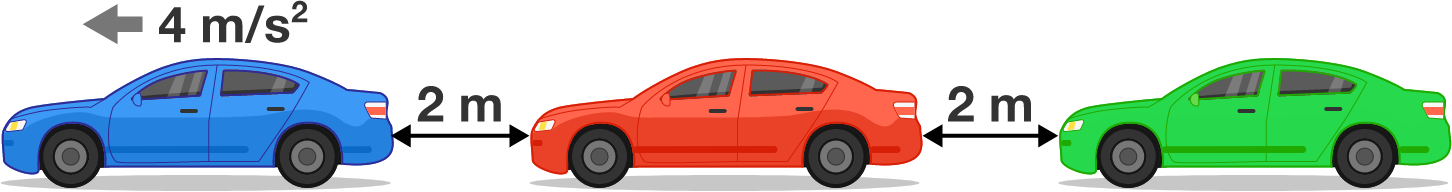# It's Green

The distance between any two adjacent cars at a red light is 2 meters.

As soon as the light turns green, the first car starts accelerating at $\SI{4}{m/s^2}.$ As soon as the first car reaches a speed of $\SI{6}{m/s},$ the second car starts accelerating at the same rate. In exactly the same fashion, each successive car starts accelerating when the car in front of it reaches $\SI{6}{m/s},$ and accelerates at $\SI{4}{m/s^2}$ until it reaches the speed $\SI{20}{m/s}.$

When all cars have reached the same speed $\SI{20}{m/s},$ how far apart are two adjacent cars (in meters)?×